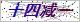网站首页  ◇  产品展示    ◇  仪表备品备件 ◇   电磁阀 > 689=118=008硅 磷表电磁阀
•••# 硅 磷表电磁阀

Z 689=118=008硅 磷表电磁阀配套与9210/9211硅、磷表使用。

Products

### 产品概述

Z689=118=008型 硅 磷表电磁阀356009，05040型 硅、磷表电磁阀O型圈Z_689=118=008硅 磷表电磁阀配套与9210/9211硅、磷表使用。

9210硅酸根/磷酸根分析仪备件

接口板        09200=A=5000
9210硅表CPU板带显示        09200=A=1000
9211磷表CPU板带显示        09200=A=1011

RS485板（MODBUS）        09125=A=0485/2485
92XX内部总线通讯板        09200=A=5500
2米内部连接电缆/4米/7米        370=302=034/304/307
921X测量板（0~1000ppb-SiO2/0~5ppm-PO4）        0921X=A=1500
9210硅表测量板（0~5000ppb-SiO2）        09210=A=1510
9211磷表测量板（0~50ppm-PO4）        09210=A=1520

O-型圈（流速调节螺钉）,4×1mm        35600905040

O-型圈（液位检测器）,38×2mm        35609935308
样水电磁阀,（溢流容器）        689=118=024
921X连接板（混合器/加热器）        09210=A=1234
921X混合器        09210=A=0400
磁性搅拌棒         226=003=013
加热元件        09097=C=0560
Pt100温度探头        35900040780
921X光缆        09210=A=0500
921X光学镜        09210=C=0340
921X镜子隔圈        09210=C=0330
O-型圈（光学镜）,22×1mm        35609905220
方形盖（光度计池）        09210=C=0310
试剂管,1,6×3,2mm        590=050=060
试剂管内插管,0,8×1,6mm
试剂泵        695=114=001
校准/冲洗泵        695=004=004
921X2升试剂桶        495=020=001
921X试剂管沉头        09210=A=0600
9210硅表化学试剂（不含硫酸）        09210=C=7000
9211磷表化学试剂（0~5ppmPO4,不含硫酸）        09211=C=7000
9211磷表化学试剂（0~50ppmPO4,不含硫酸）        09211=C=7001
9210硅表药剂（一年用量）        09210=A=0507

9210硅表测量槽清洗

1.用一字螺丝刀拧下测量槽正面的螺丝盖，将光学镜和垫片沿边沿取下，小心不要划伤镜面；
2.用除盐水清洗光学镜和小磁棒。若镜面上有油状物，可用洁净软布轻轻擦试；
3.进入仪表维护菜单下的电磁阀子菜单。打开阀1-6中有水样的电磁阀和7＃阀，使水样流入测量，便可以达到清洗测量槽的目的了；
4.将清洗好的光学镜和垫片(注意垫片方向)以及小磁棒放回测量槽，这样清洗工作完成；
5.如果还是清洗不干浄，可以打开上面的测量槽盖子，用除盐水多次清洗就可以了，如果还是有不干净，可以用草酸清洗，小磁棒上好不要是黑色的，如果变黑了就把黑色刮掉就可以了；
6.一般泵不动作都是泵本身的问题，比如堵塞。你可以用10％的氨水清洗下

合肥超纯仪表科技有限公司是从事于火电、核电、污水处理厂、冶炼、环保、钢铁厂、水泥厂、供热厂等领域所需实验室超纯水器、水处理系统、发电机定冷水改造、电厂化学仪表维护等化学设备的专业研发、生产和销售厂家。

### 留言框

• #### 验证码：请输入计算结果（填写阿拉伯数字），如：三加四=7

13865908184